# 1.本章学习总结（2分）

## 1.1 学习内容总结

• 结构体的定义
struct  结构名
{
变量类型 变量名;
变量类型 变量名;
...
};

• 结构体成员赋值
结构名.变量名=赋值内容;
or


• 结构体数组排序做法
void sort(struct stu* p, int n)
{
int i, j;
struct stu temp;
for(i=0;i<n-1;i++)
{
for (j = 0; j < n - i - 1; j++)
{
if (p[j].score < p[j + 1].score)
{
temp = p[j];
p[j] = p[j + 1];
p[j + 1] = temp;
}
}
}
}


• 结构体指针怎么用

struct student
{
char name;
int score;
};
struct student*p;
or
struct student
{
char name;
int score;
}*p;


整体调用：
stu1=*p;

p->score=100;

• 共用体、枚举类型做法

union Data
{
int i;
float f;
char  str;
} data;


enum DAY
{
MON=1, TUE, WED, THU, FRI, SAT, SUN
};

• 文件读写，文件中数据读进结构体数组

fp=fopen("abc.txt"."r+");


while(!feof(fp))
{
fscanf(fp, "%d,%s,%d", &stu[i].num, &stu[i].name, &stu[i]. score);
}


# 2.综合作业--“我爱成语”

## 2.1.文件介绍

### 1.头文件介绍

• idiom.h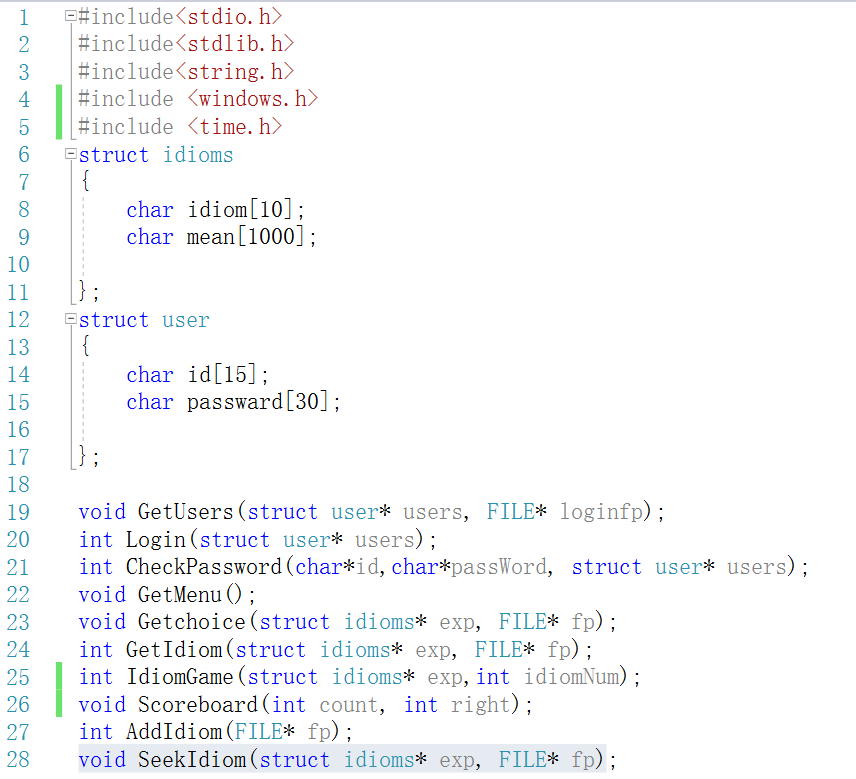### 2.函数实现文件介绍。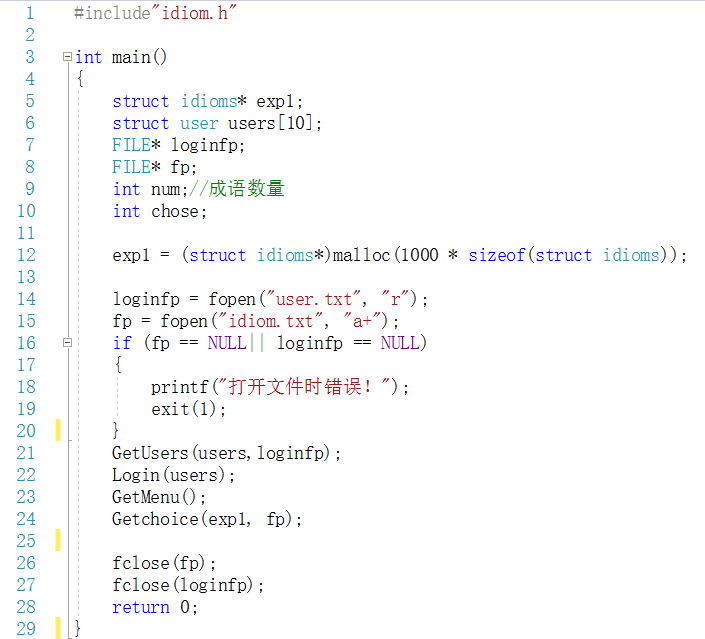• 函数声明1：void GetUsers(struct user* users, FILE* loginfp)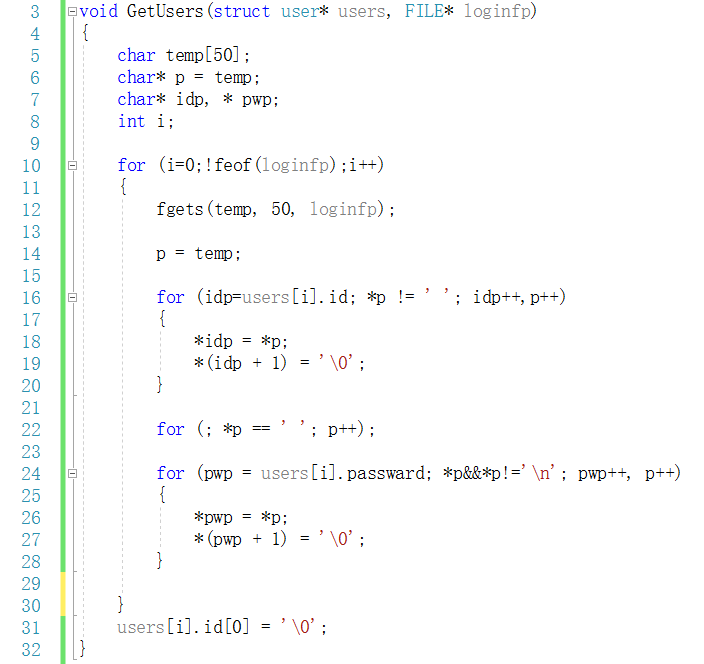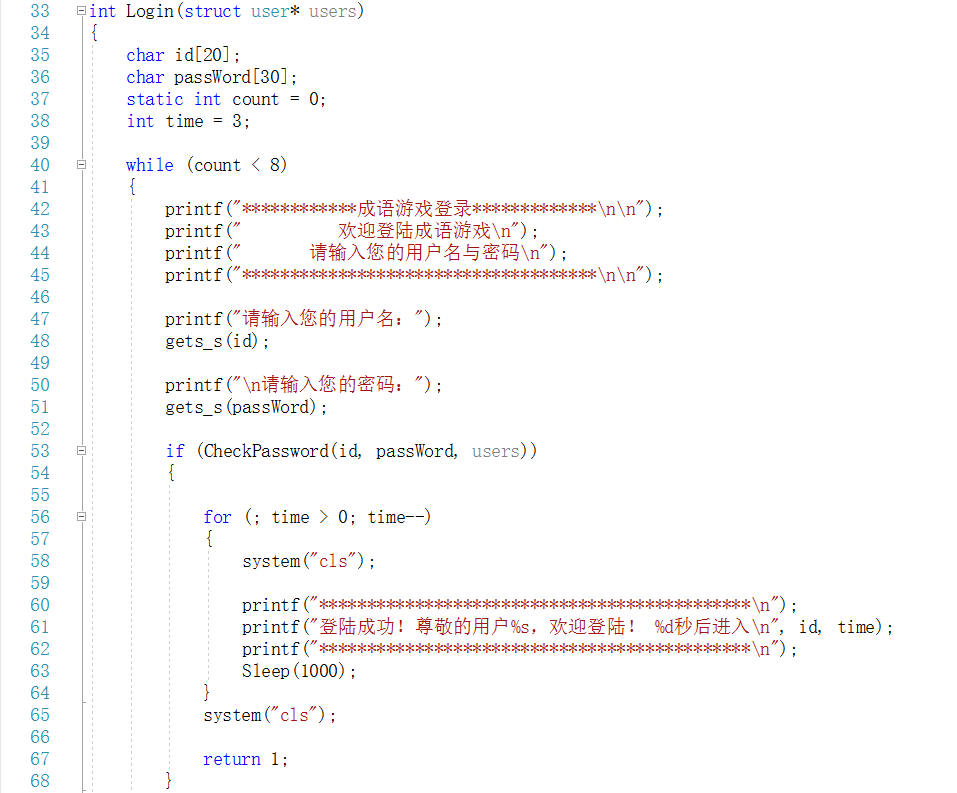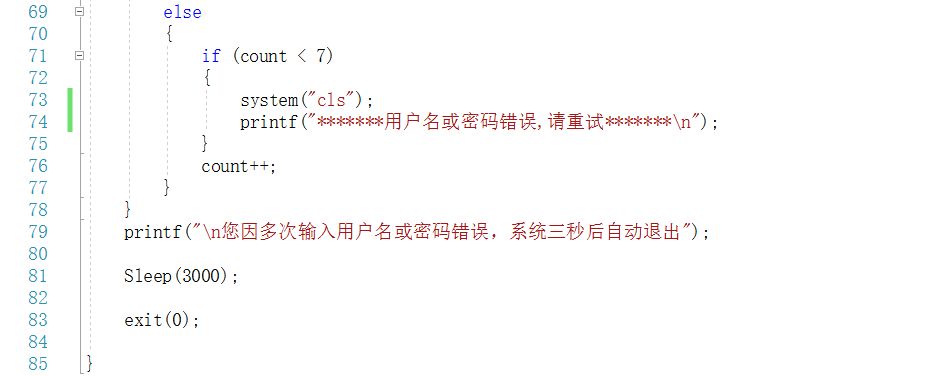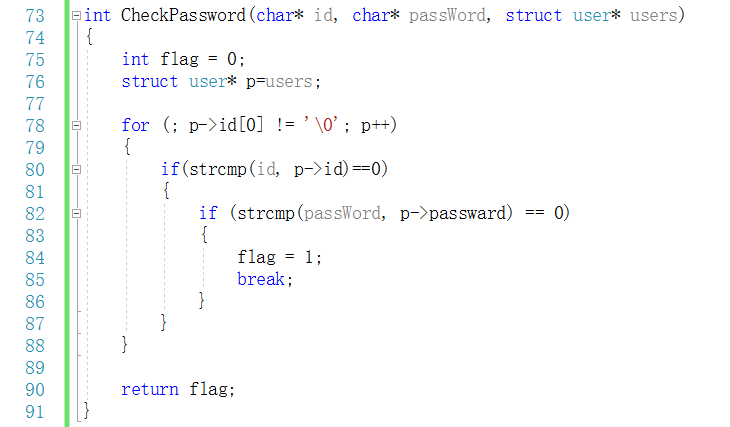• 函数声明1：void GetMenu(struct idioms* exp, FILE* fp)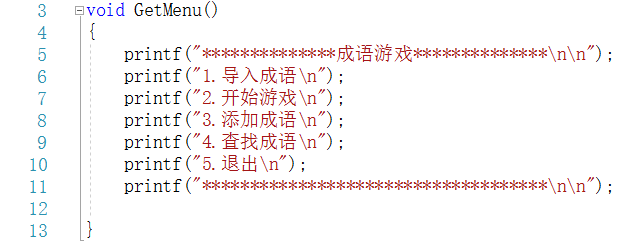• 函数声明2：void Getchoice(struct idioms* exp, FILE* fp)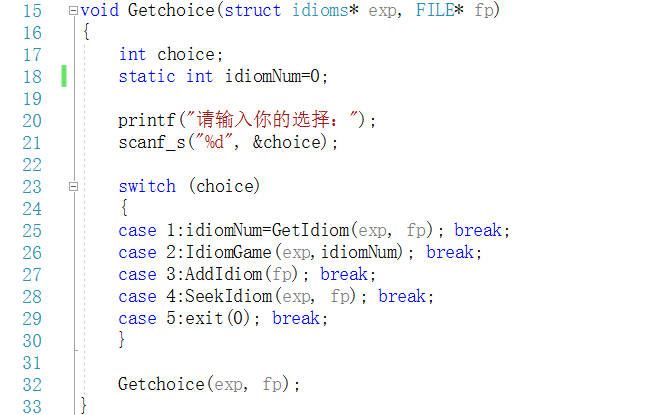• 函数声明3：int GetIdiom(struct idioms* exp, FILE* fp)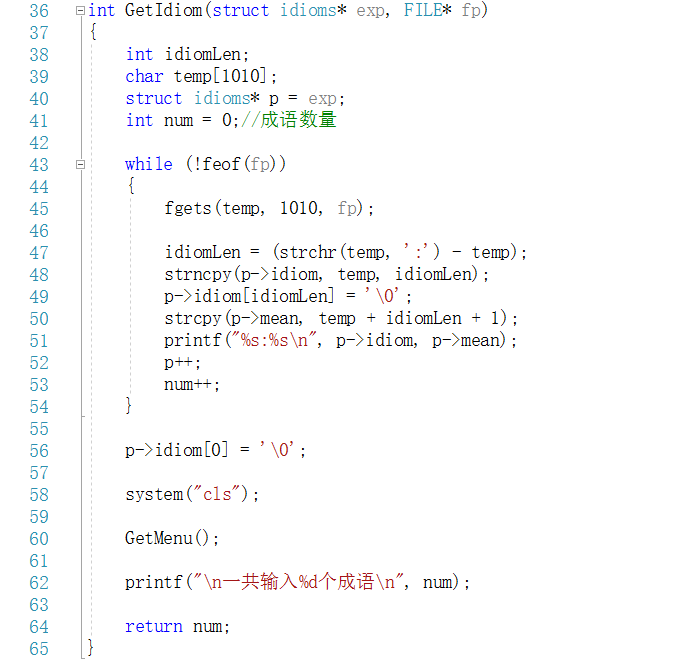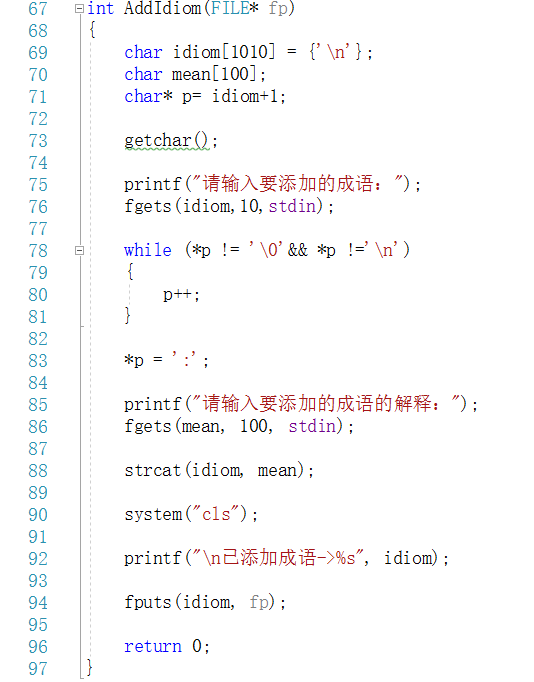• 函数声明5：void SeekIdiom(struct idioms* exp, FILE* fp)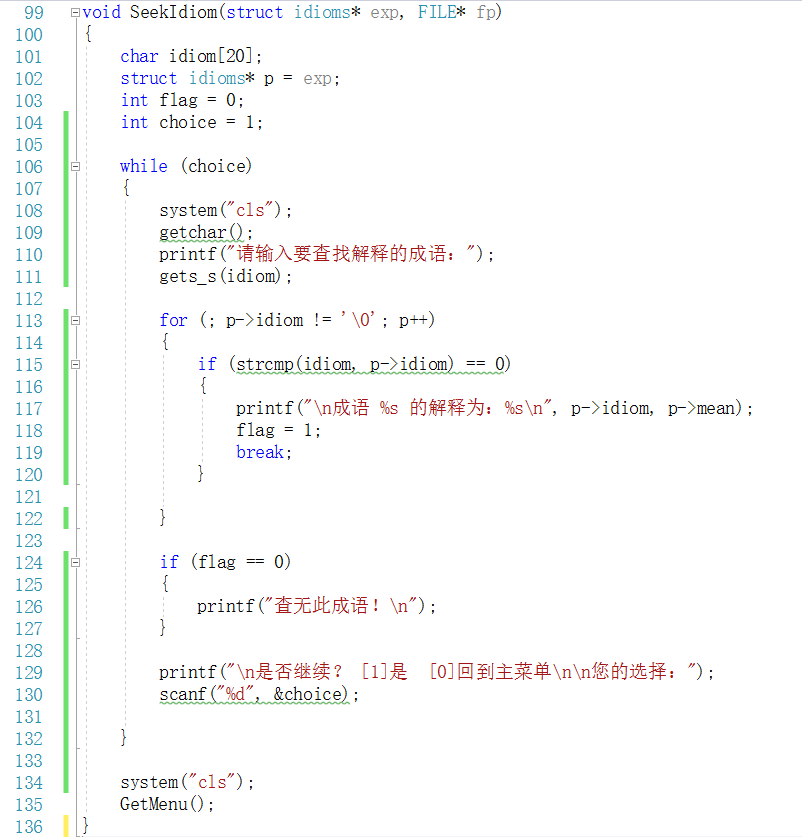• 函数声明1：int IdiomGame(struct idioms* exp, int idiomNum)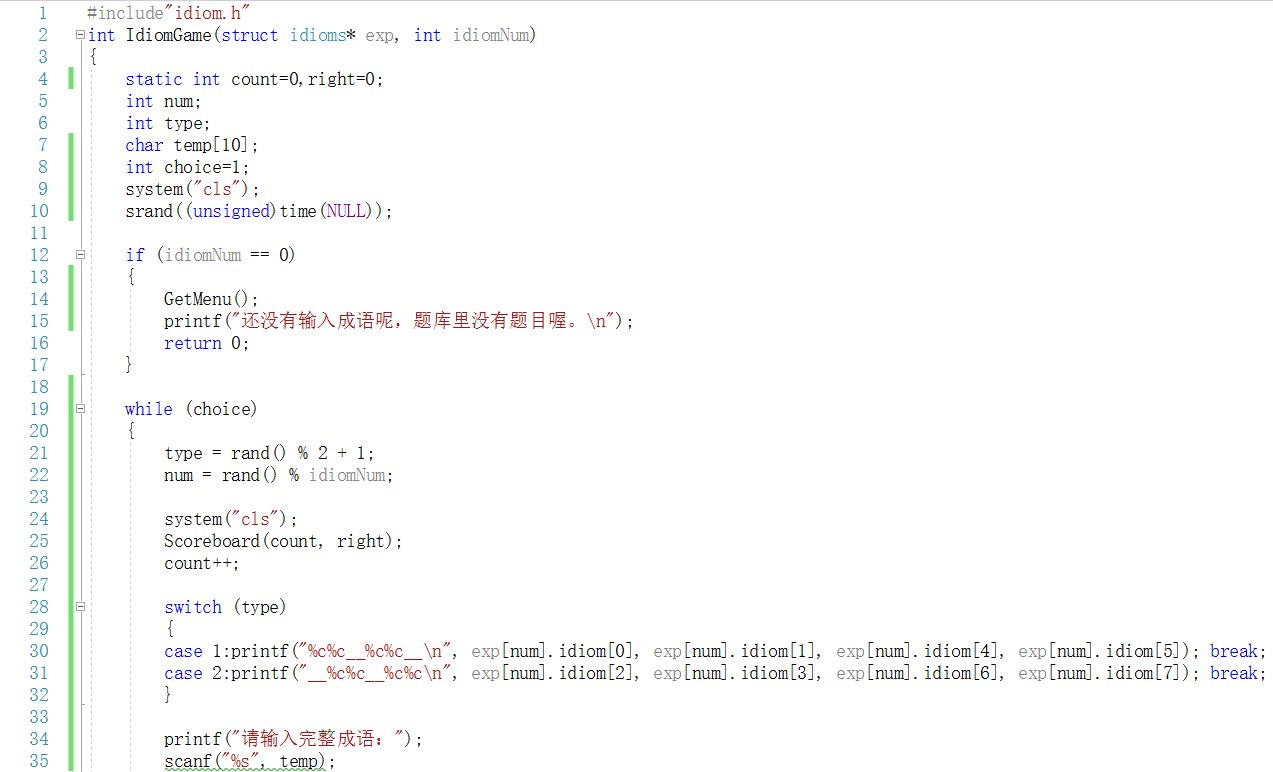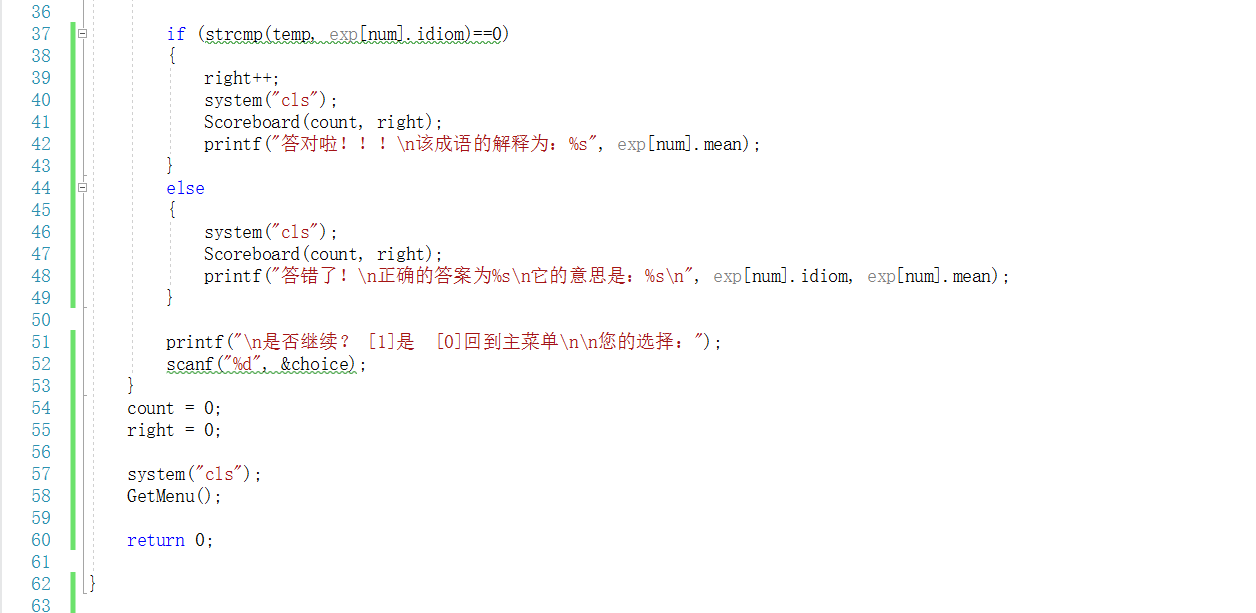• 函数声明2：void Scoreboard(int count, int right)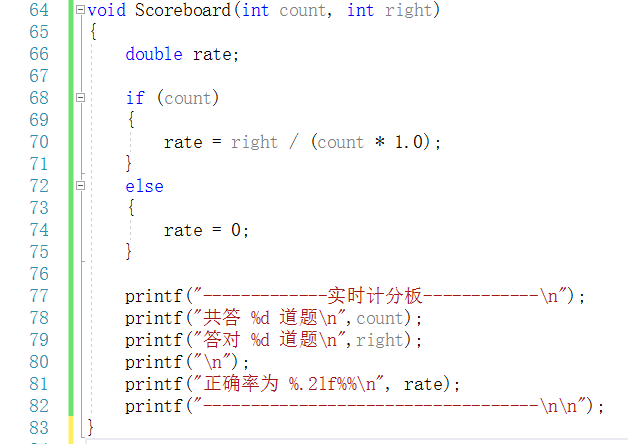## 2.2.运行结果

### 1.登录界面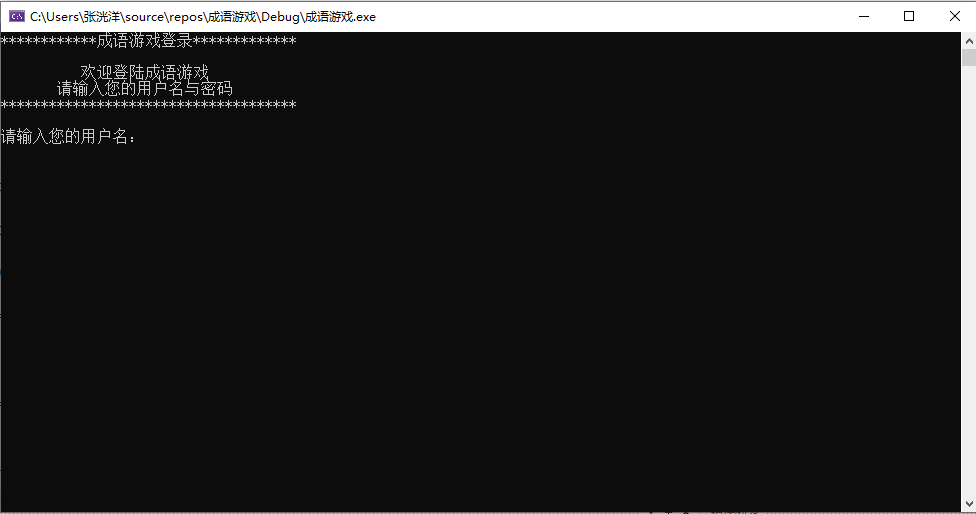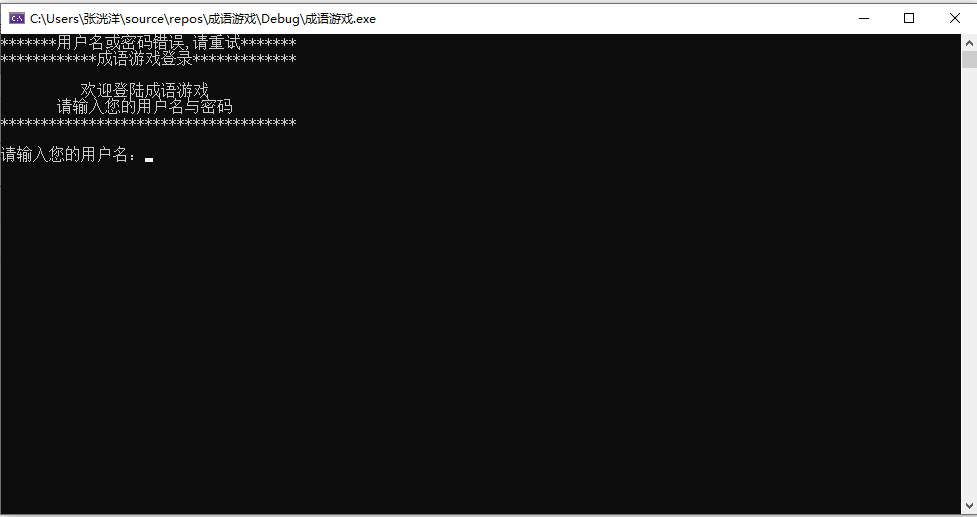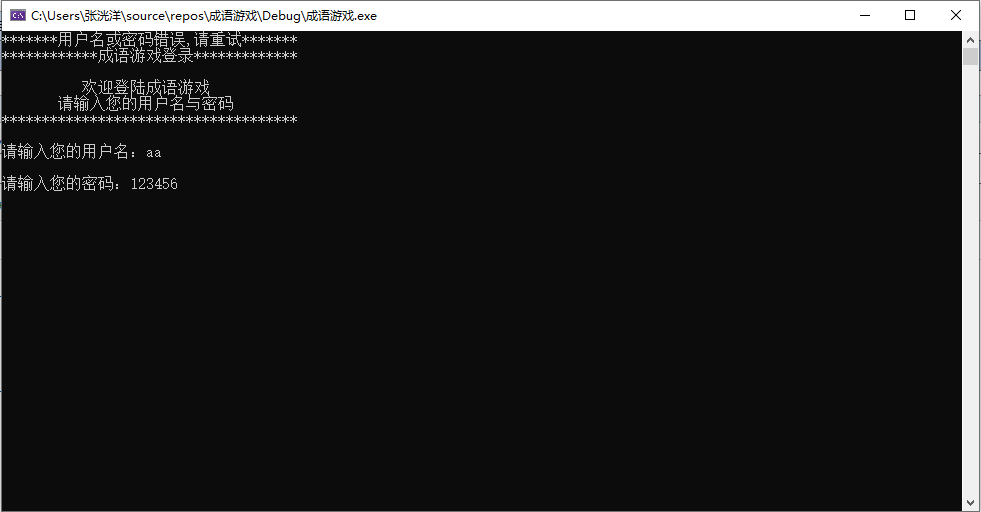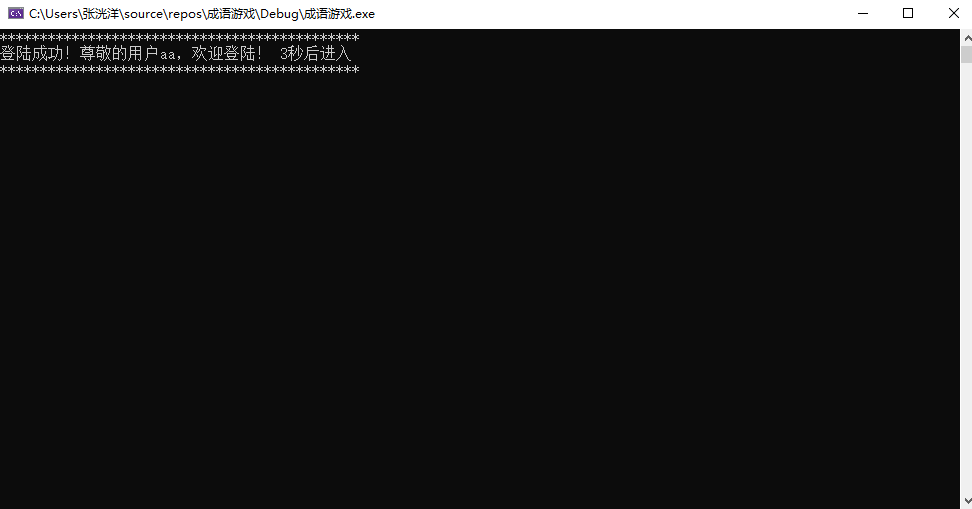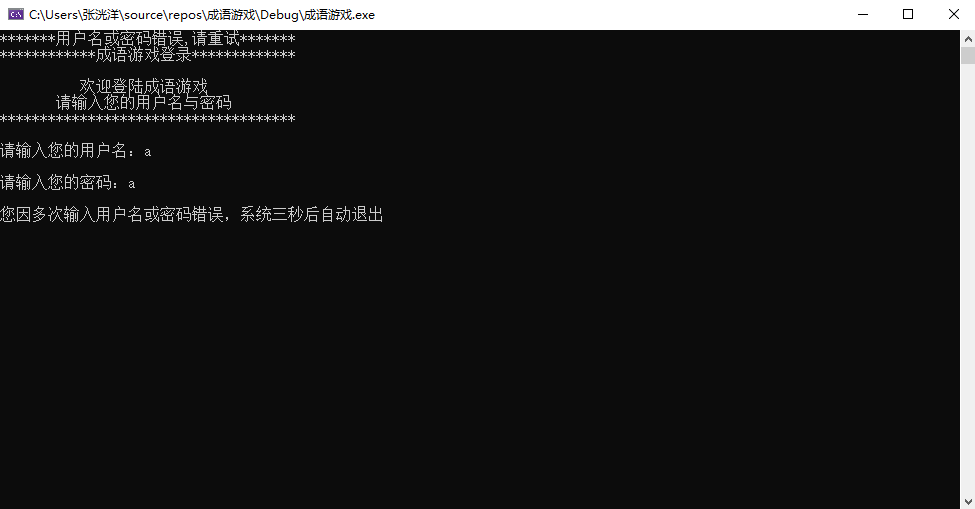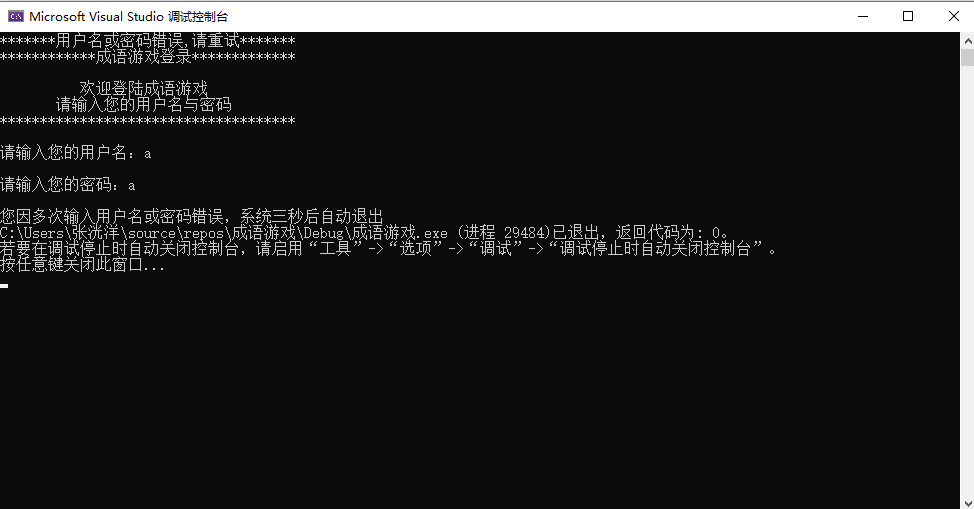### 2.游戏界面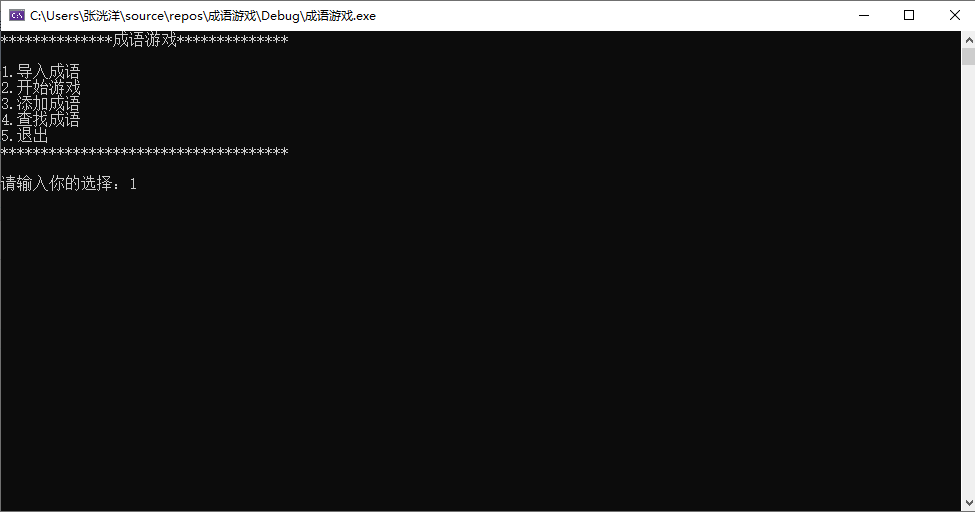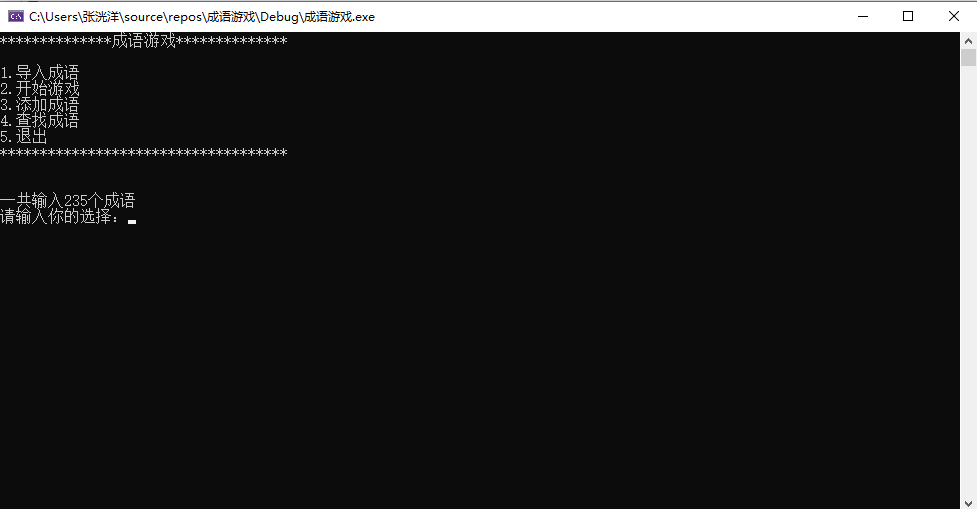（如果不导入就开始）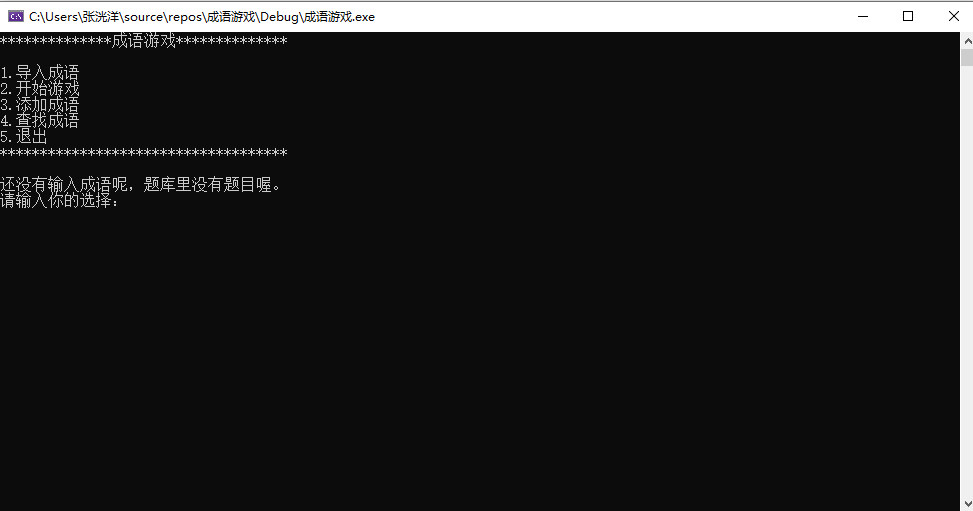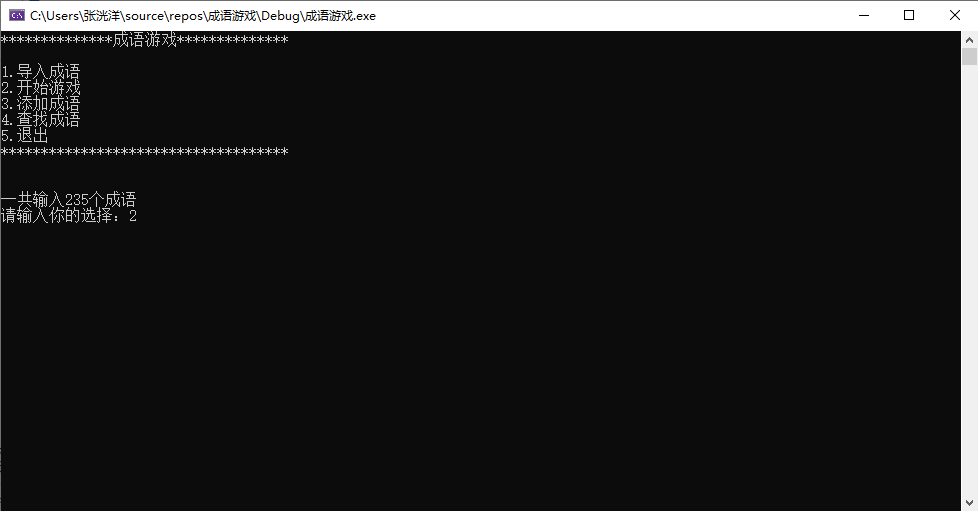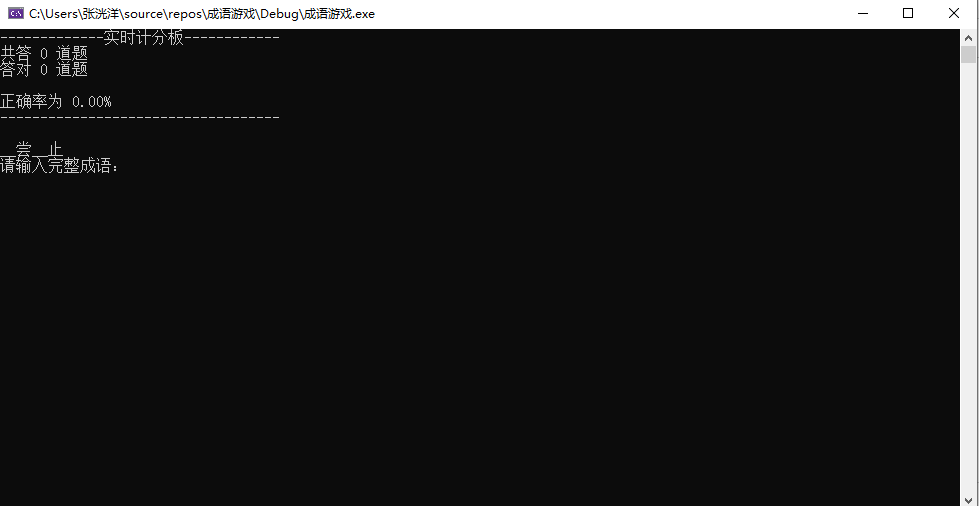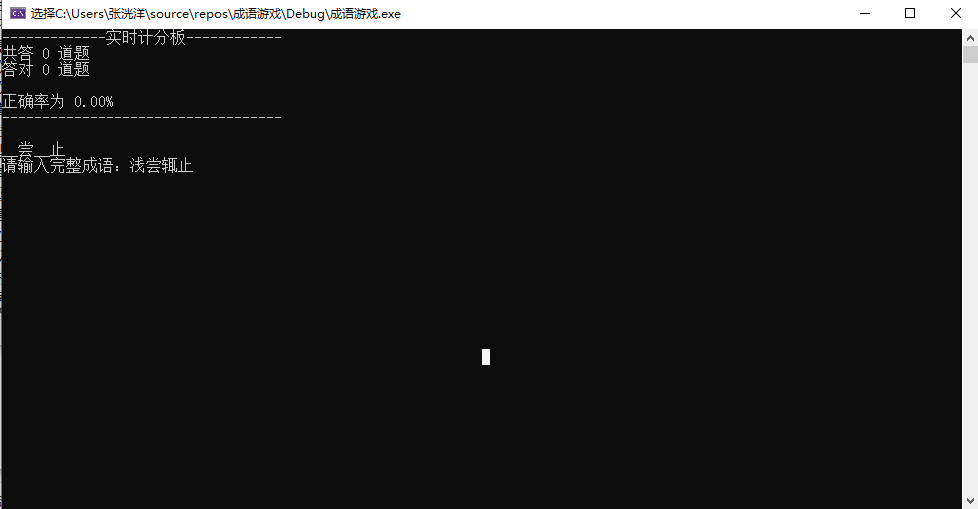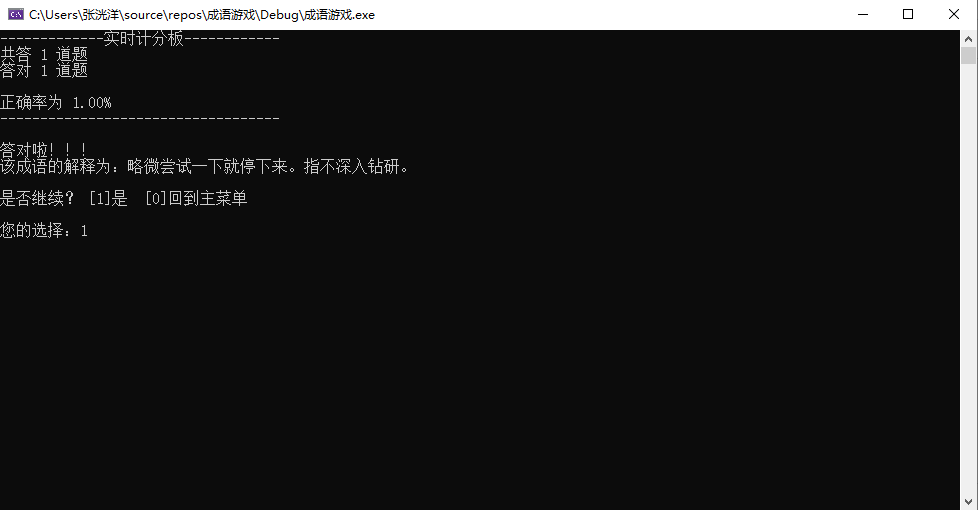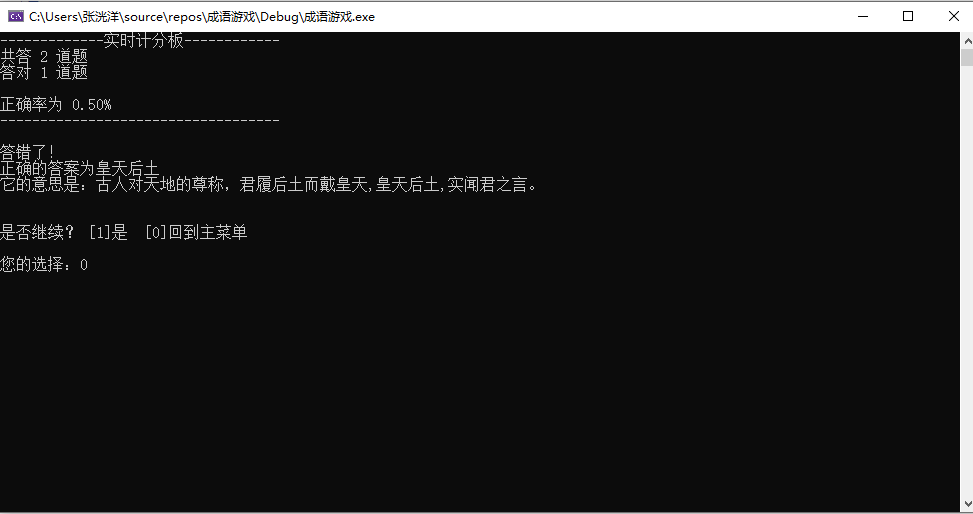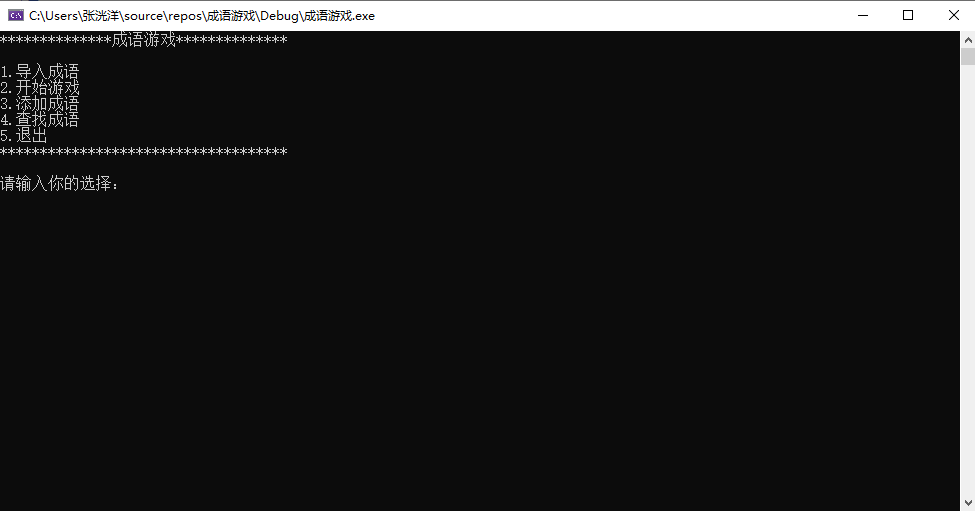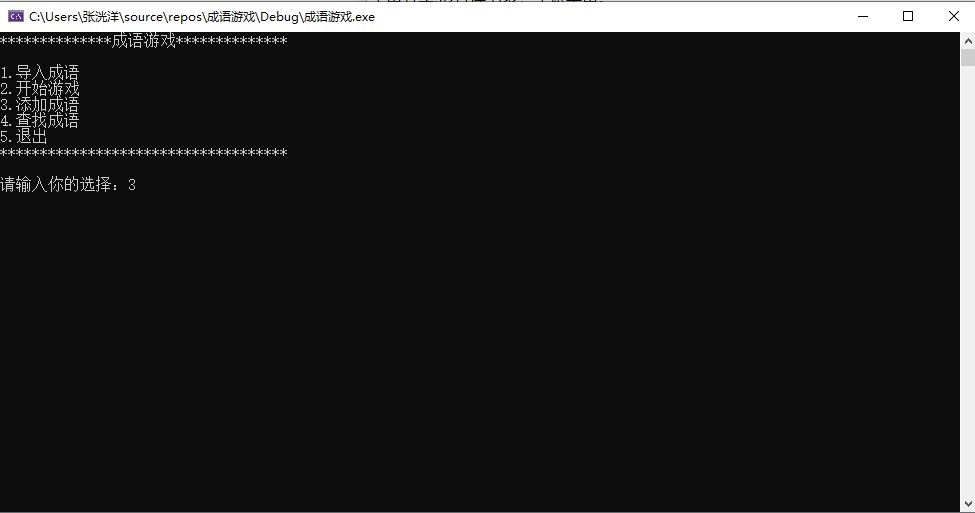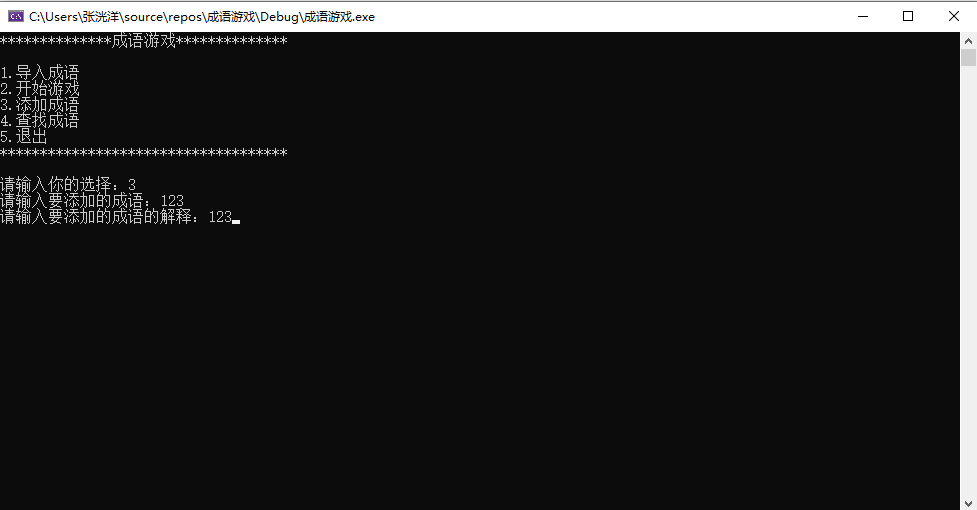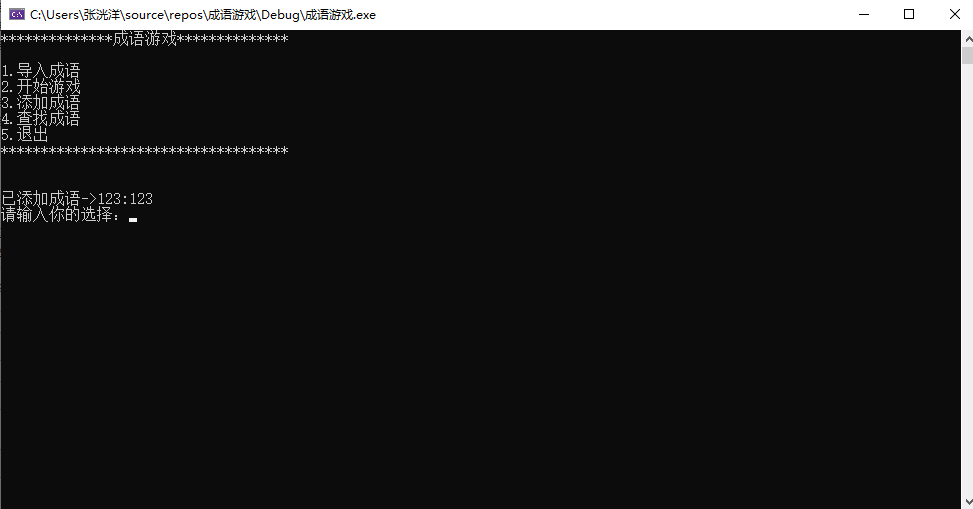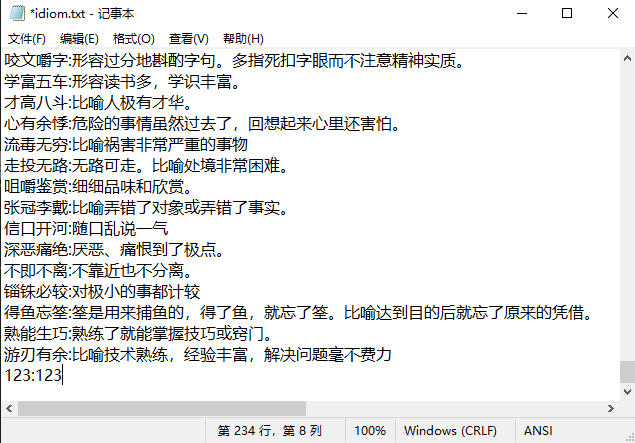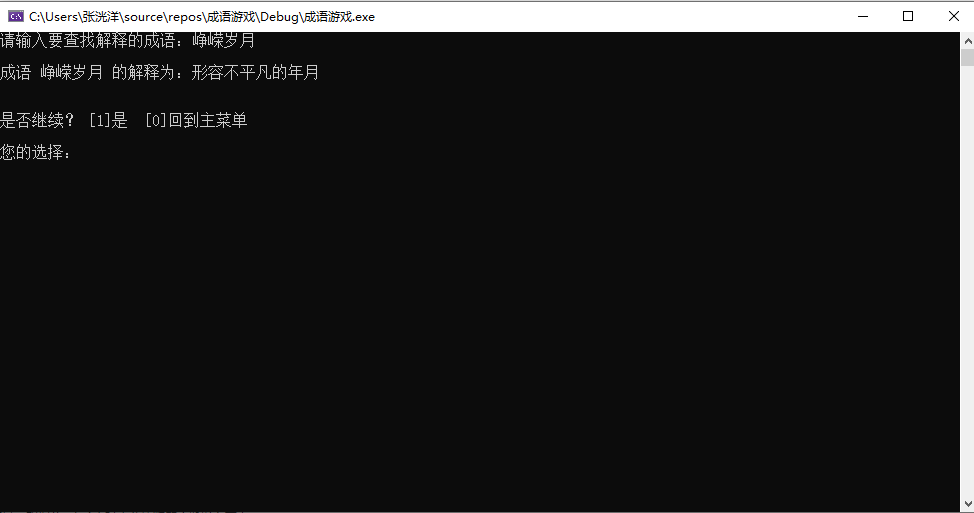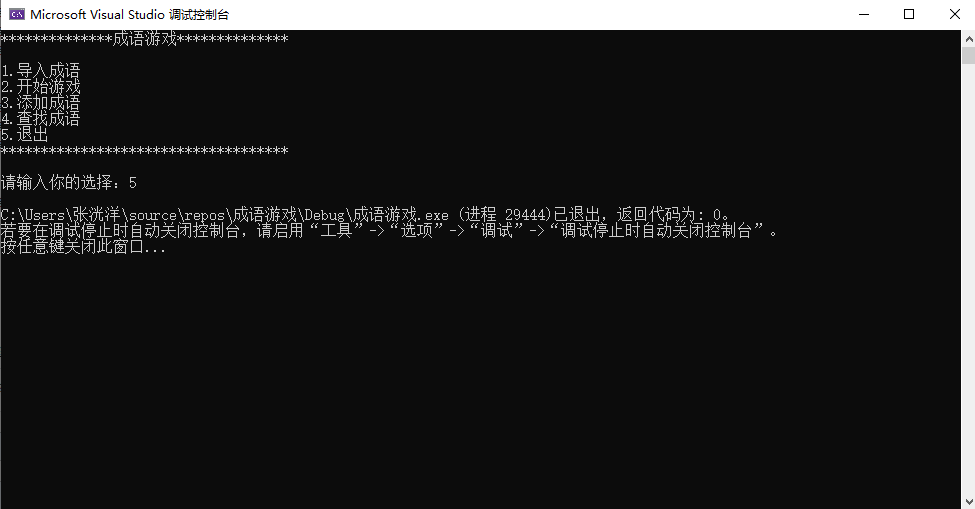## 2.3大作业总结

posted @ 2019-12-15 22:16  ZGGYY  阅读(238)  评论(2编辑  收藏  举报